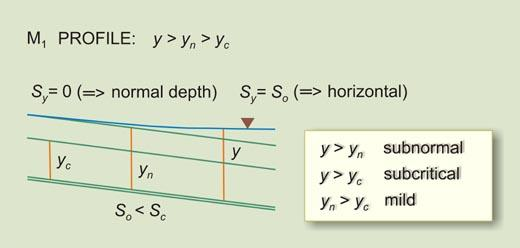online_wsprofiles_21:   M1 water-surface profileDESCRIPTION
General

• The php script [program]   online_wsprofiles_21   calculates an M1 water-surface profile in a prismatic open channel using the direct-step method (Chow, V. T.: Open-channel hydraulics, McGraw-Hill, 1959).

• The program calculates normal depth based on the geometric and hydraulic input data. Newton's iteration is used to solve the nonlinear equations.

• An M1 profile is subnormal, subcritical, and mild (see figure). The calculated gradually varied flow depth varies between the specified flow depth at the downstream boundary and [it is asymptotic to] normal depth upstream.

Input

• There are five required input variables:   discharge Q, bottom width B, side slope z, bottom slope So, and Manning's n.

• For the downstream boundary condition, you can specify a suitable input flow depth. Alternatively, if the input flow depth box is left blank, the program will use a value close to normal depth (1.01 * yn) instead.

• The program echoes the input data. Internally calculated normal depth is echoed with five-decimal (5) accuracy.

Defaults

• For the input flow depth, you can enter any suitable [subcritical] subnormal flow depth, i.e., a flow depth greater than normal depth. Leave this box blank if you want the program to use a value close to normal depth (1.01 * yn) [In general, this option is not recommended, because the M1 profile would be very close to uniform flow].

• You can enter the desired number of computational intervals n. The suggested range is 50-200. A higher number means higher computational accuracy. Leave this box blank if you want the program to set the default value at 100.

• You can enter the desired number of tabular output intervals m. The suggested range is 10-100. A higher number means more detailed [longer] output. Leave this box blank if you want the program to set the default value at 10.

Output

• A table with thirteen (13) columns: output interval index k, [flow] depth (m), [flow] area (m2), velocity (m/s), velocity head (m), specific head (m), wetted perimeter (m), hydraulic radius (m), friction slope (m/m), average [friction] slope (m/m), specific head difference (m), length increment (m), and total [channel] length (m).

• The number of computational intervals n and the number of tabular output intervals m are printed for reference.

• The calculated normal depth and normal-depth Froude number are printed for reference. If the normal-depth Froude number exceeds 0.99, execution is halted (see error message No. 7 below).

Units

• The current version is written in SI [metric] units.

Errors

• Enter only positive [nonzero] numbers for discharge, Manning's n, and bottom slope. Failure to do this will trigger an error message and halt execution (Error messages No. 1: A, B, and C, respectively).

• Enter only nonnegative [zero or greater] numbers for bottom width and side slope. Failure to do this will trigger an error message and halt execution (Error messages No. 2: A and B, respectively).

• Bottom width and side slope cannot be zero at the same time. Failure to do this will trigger an error message and halt execution (Error message No. 3).

• A Manning's n value outside of the range 0.008-0.250 will trigger an error message and halt execution (Error message No. 4).

• A bottom slope outside of the range 0.000005-0.05 will trigger an error message and halt execution (Error message No. 5).

• An input flow depth less than or equal to the calculated normal depth will trigger an error message and halt execution (Error message No. 6).

• A normal-depth Froude number greater than 0.99 will trigger an error message and halt execution (Error message No. 7).

Thank you for running online_wsprofiles_21.   Please call again.   [Version 1.0.0,   070430]